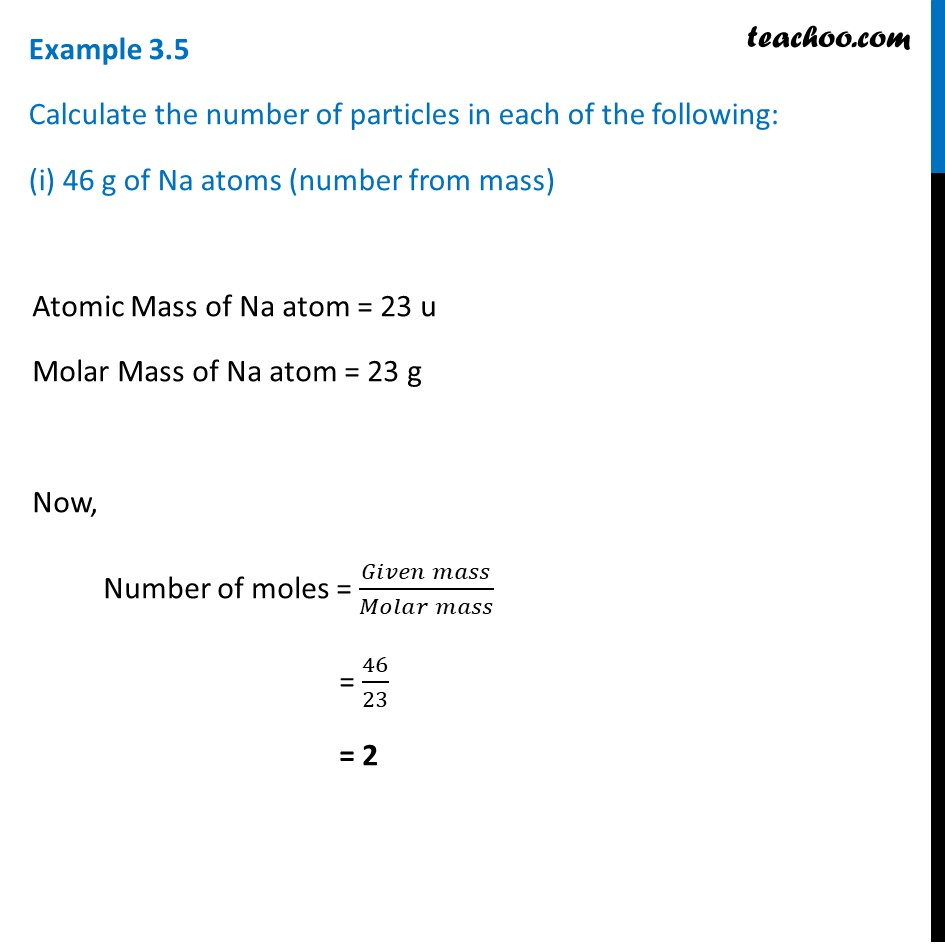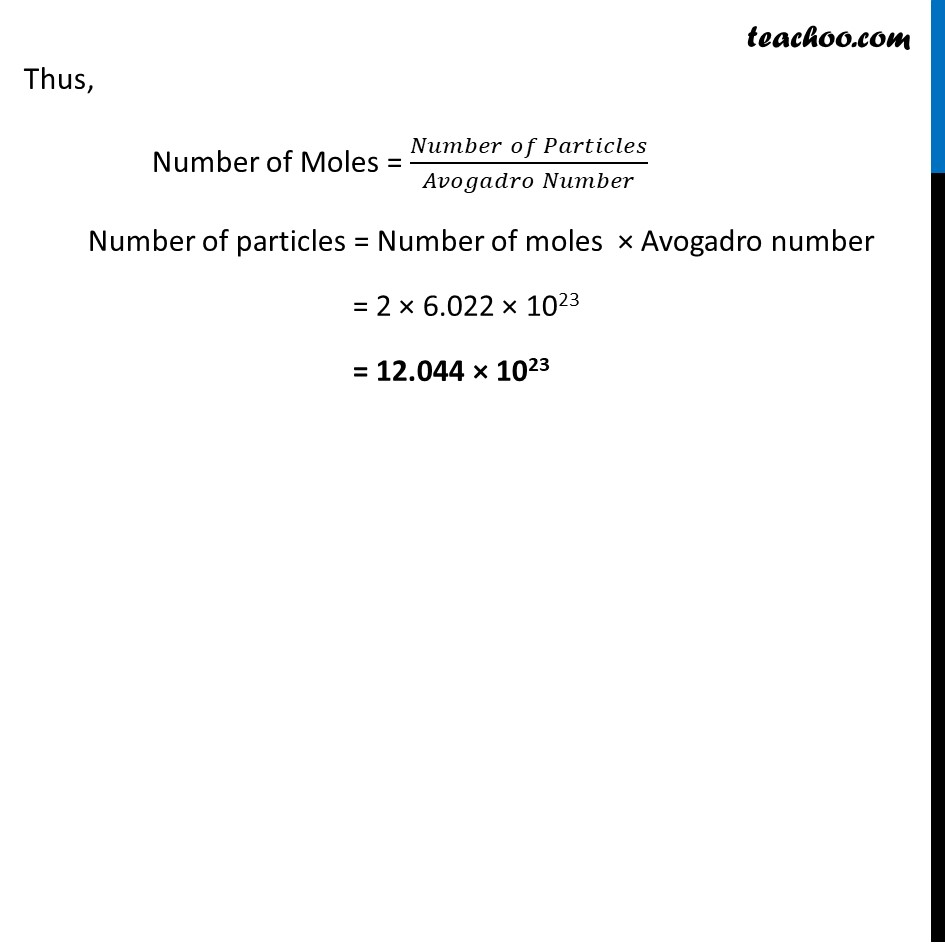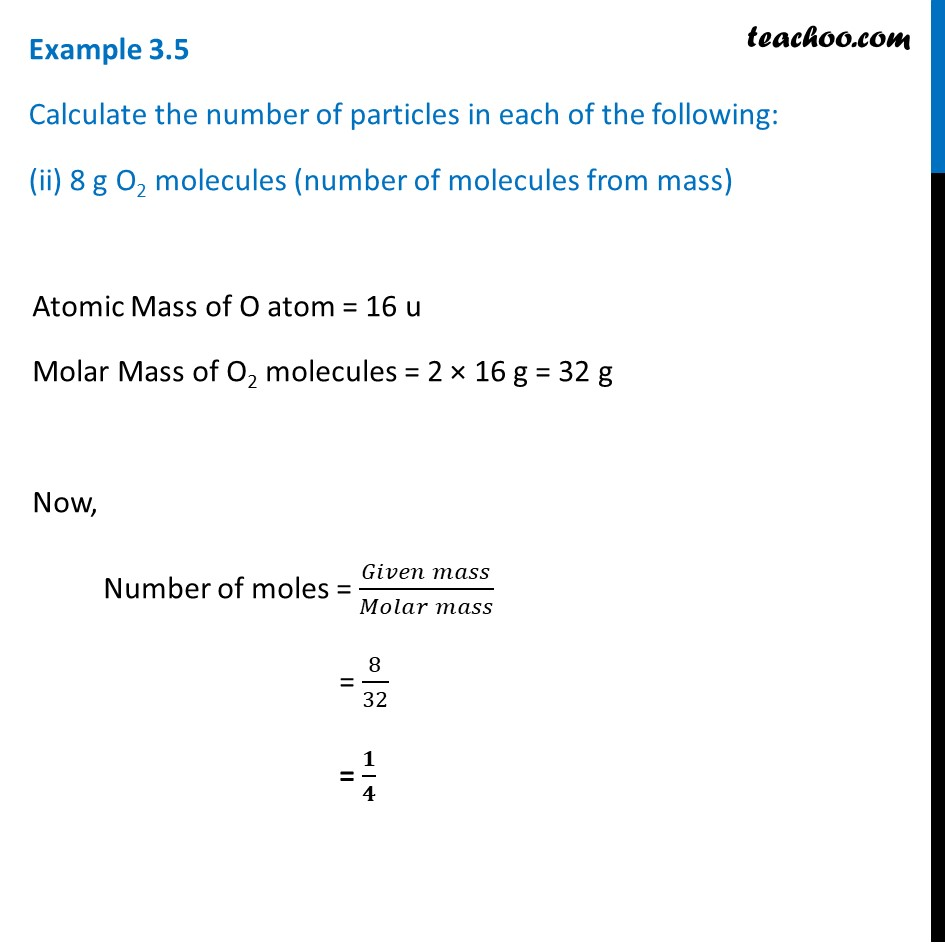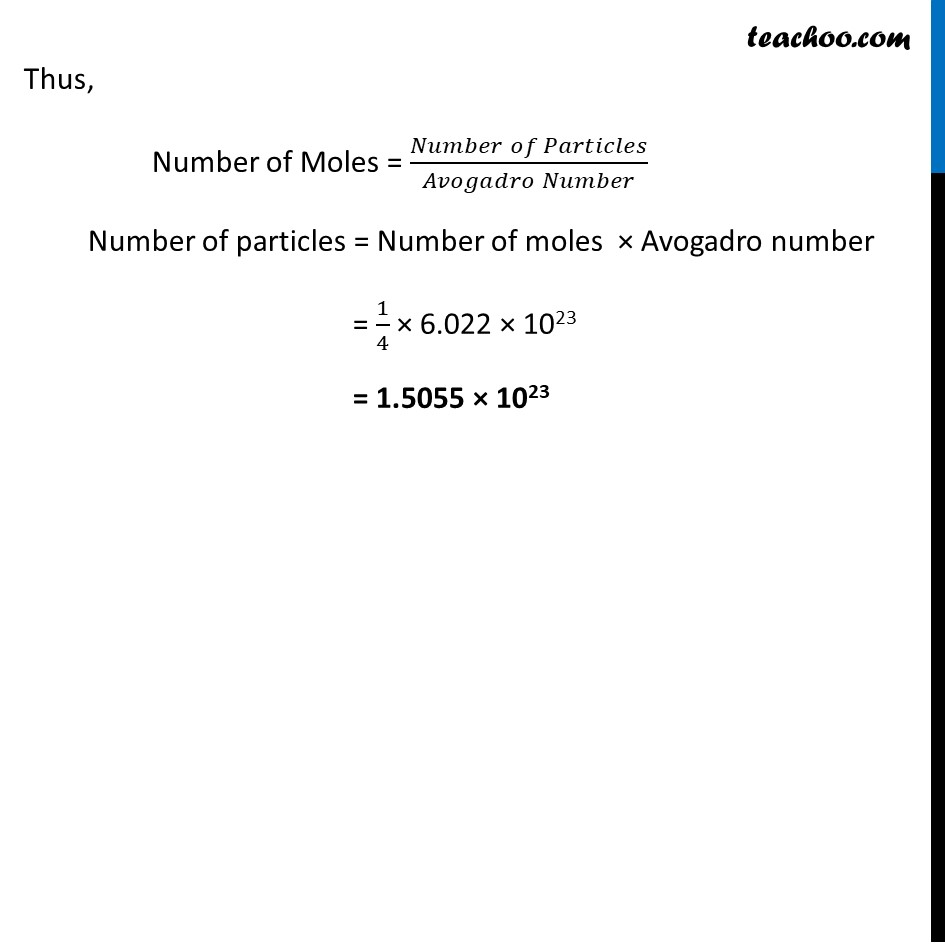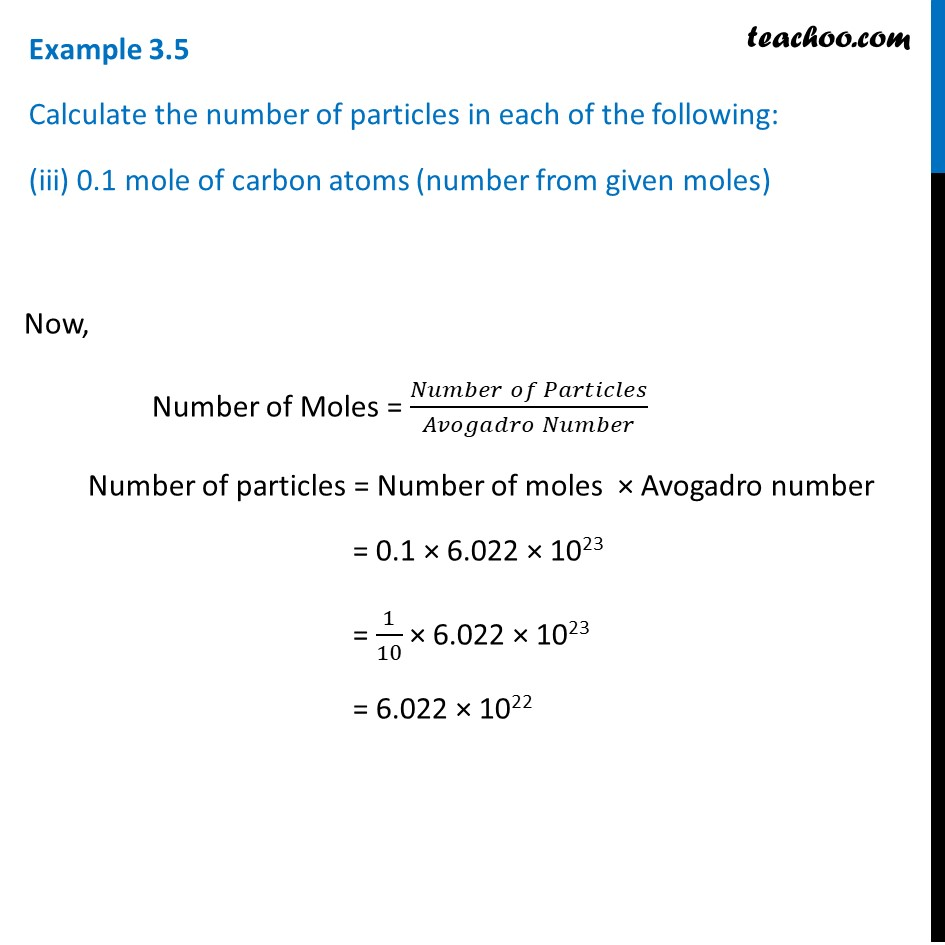1. Class 9
2. Chapter 3 Class 9 - Atoms And Molecules (Term 2)
3. Examples from NCERT Book

Transcript

Example 3.5 Calculate the number of particles in each of the following: (i) 46 g of Na atoms (number from mass) Atomic Mass of Na atom = 23 u Molar Mass of Na atom = 23 g Now, Number of moles = (𝐺𝑖𝑣𝑒𝑛 𝑚𝑎𝑠𝑠)/(𝑀𝑜𝑙𝑎𝑟 𝑚𝑎𝑠𝑠) = 46/23 = 2 Thus, Number of Moles = (𝑁𝑢𝑚𝑏𝑒𝑟 𝑜𝑓 𝑃𝑎𝑟𝑡𝑖𝑐𝑙𝑒𝑠)/(𝐴𝑣𝑜𝑔𝑎𝑑𝑟𝑜 𝑁𝑢𝑚𝑏𝑒𝑟) Number of particles = Number of moles × Avogadro number = 2 × 6.022 × 1023 = 12.044 × 1023 Example 3.5 Calculate the number of particles in each of the following: (ii) 8 g O2 molecules (number of molecules from mass) Atomic Mass of O atom = 16 u Molar Mass of O2 molecules = 2 × 16 g = 32 g Now, Number of moles = (𝐺𝑖𝑣𝑒𝑛 𝑚𝑎𝑠𝑠)/(𝑀𝑜𝑙𝑎𝑟 𝑚𝑎𝑠𝑠) = 8/32 = 𝟏/𝟒 Thus, Number of Moles = (𝑁𝑢𝑚𝑏𝑒𝑟 𝑜𝑓 𝑃𝑎𝑟𝑡𝑖𝑐𝑙𝑒𝑠)/(𝐴𝑣𝑜𝑔𝑎𝑑𝑟𝑜 𝑁𝑢𝑚𝑏𝑒𝑟) Number of particles = Number of moles × Avogadro number = 1/4 × 6.022 × 1023 = 1.5055 × 1023 Example 3.5 Calculate the number of particles in each of the following: (iii) 0.1 mole of carbon atoms (number from given moles) Now, Number of Moles = (𝑁𝑢𝑚𝑏𝑒𝑟 𝑜𝑓 𝑃𝑎𝑟𝑡𝑖𝑐𝑙𝑒𝑠)/(𝐴𝑣𝑜𝑔𝑎𝑑𝑟𝑜 𝑁𝑢𝑚𝑏𝑒𝑟) Number of particles = Number of moles × Avogadro number = 0.1 × 6.022 × 1023 = 1/10 × 6.022 × 1023 = 6.022 × 1022

Examples from NCERT Book

Class 9
Chapter 3 Class 9 - Atoms And Molecules (Term 2)